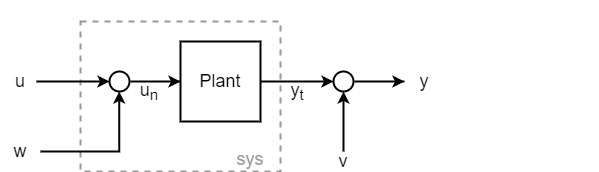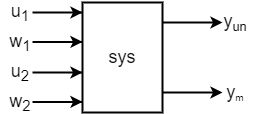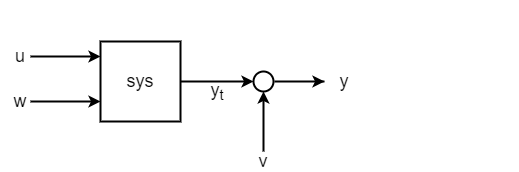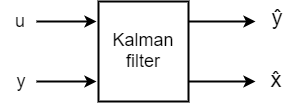# kalman

Design Kalman filter for state estimation

## Syntax

``[kalmf,L,P] = kalman(sys,Q,R,N)``
``[kalmf,L,P] = kalman(sys,Q,R,N,sensors,known)``
``[kalmf,L,P,Mx,Z,My] = kalman(___)``
``[kalmf,L,P,Mx,Z,My] = kalman(___,type)``

## Description

example

````[kalmf,L,P] = kalman(sys,Q,R,N)` creates a Kalman filter given the plant model `sys` and the noise covariance data `Q`, `R`, and `N`. The function computes a Kalman filter for use in a Kalman estimator with the configuration shown in the following diagram.You construct the model `sys` with known inputs u and white process noise inputs w, such that w consists of the last Nw inputs to `sys`. The "true" plant output yt consists of all outputs of `sys`. You also provide the noise covariance data `Q`, `R`, and `N`. The returned Kalman filter `kalmf` is a state-space model that takes the known inputs u and the noisy measurements y and produces an estimate $\stackrel{^}{y}$ of the true plant output and an estimate $\stackrel{^}{x}$ of the plant states. `kalman` also returns the Kalman gains `L` and the steady-state error covariance matrix `P`. ```

example

````[kalmf,L,P] = kalman(sys,Q,R,N,sensors,known)` computes a Kalman filter when one or both of the following conditions exist. Not all outputs of `sys` are measured.The disturbance inputs w are not the last inputs of `sys`. The index vector `sensors` specifies which outputs of `sys` are measured. These outputs make up y. The index vector `known` specifies which inputs are known (deterministic). The known inputs make up u. The `kalman` command takes the remaining inputs of `sys` to be the stochastic inputs w.```
````[kalmf,L,P,Mx,Z,My] = kalman(___)` also returns the innovation gains `Mx` and `My` and the steady-state error covariances `P` and `Z` for a discrete-time `sys`. You can use this syntax with any of the previous input argument combinations. ```
````[kalmf,L,P,Mx,Z,My] = kalman(___,type)` specifies the estimator type for a discrete-time `sys`. `type = 'current'` — Compute output estimates $\stackrel{^}{y}\left[n|n\right]$ and state estimates $\stackrel{^}{x}\left[n|n\right]$ using all available measurements up to $y\left[n\right]$.`type = 'delayed'` — Compute output estimates $\stackrel{^}{y}\left[n|n-1\right]$ and state estimates $\stackrel{^}{x}\left[n|n-1\right]$ using measurements only up to $y\left[n-1\right]$. The delayed estimator is easier to implement inside control loops. You can use the `type` input argument with any of the previous input argument combinations.```

## Examples

collapse all

Design a Kalman filter for a plant that has additive white noise w on the input and v on the output, as shown in the following diagram.Assume that the plant has the following state-space matrices and is a discrete-time plant with an unspecified sample time (`Ts = -1`).

```A = [1.1269 -0.4940 0.1129 1.0000 0 0 0 1.0000 0]; B = [-0.3832 0.5919 0.5191]; C = [1 0 0]; D = 0; Plant = ss(A,B,C,D,-1); Plant.InputName = 'un'; Plant.OutputName = 'yt';```

To use `kalman`, you must provide a model `sys` that has an input for the noise `w`. Thus, `sys` is not the same as `Plant`, because `Plant` takes the input `un = u + w`. You can construct `sys` by creating a summing junction for the noise input.

```Sum = sumblk('un = u + w'); sys = connect(Plant,Sum,{'u','w'},'yt');```

Equivalently, you can use `sys = Plant*[1 1]`.

Specify the noise covariances. Because the plant has one noise input and one output, these values are scalar. In practice, these values are properties of the noise sources in your system, which you determine by measurement or other knowledge of your system. For this example, assume both noise sources have unit covariance and are not correlated (`N` = 0).

```Q = 1; R = 1; N = 0;```

Design the filter.

```[kalmf,L,P] = kalman(sys,Q,R,N); size(kalmf)```
```State-space model with 4 outputs, 2 inputs, and 3 states. ```

The Kalman filter `kalmf` is a state-space model having two inputs and four outputs. `kalmf` takes as inputs the plant input signal u and the noisy plant output $y={y}_{t}+v$. The first output is the estimated true plant output $\underset{}{\overset{ˆ}{y}}$. The remaining three outputs are the state estimates $\underset{}{\overset{ˆ}{x}}$. Examine the input and output names of `kalmf` to see how `kalman` labels them accordingly.

`kalmf.InputName`
```ans = 2x1 cell {'u' } {'yt'} ```
`kalmf.OutputName`
```ans = 4x1 cell {'yt_e'} {'x1_e'} {'x2_e'} {'x3_e'} ```

Examine the Kalman gains `L`. For a SISO plant with three states, `L` is a three-element column vector.

`L`
```L = 3×1 0.3586 0.3798 0.0817 ```

For an example that shows how to use `kalmf` to reduce measurement error due to noise, see Kalman Filtering.

Consider a plant with three inputs, one of which represents process noise w, and two measured outputs. The plant has four states.Assuming the following state-space matrices, create `sys`.

```A = [-0.71 0.06 -0.19 -0.17; 0.06 -0.52 -0.03 0.30; -0.19 -0.03 -0.24 -0.02; -0.17 0.30 -0.02 -0.41]; B = [ 1.44 2.91 0; -1.97 0.83 -0.27; -0.20 1.39 1.10; -1.2 0 -0.28]; C = [ 0 -0.36 -1.58 0.28; -2.05 0 0.51 0.03]; D = zeros(2,3); sys = ss(A,B,C,D); sys.InputName = {'u1','u2','w'}; sys.OutputName = {'y1','y2'};```

Because the plant has only one process noise input, the covariance Q is a scalar. For this example, assume the process noise has unit covariance.

`Q = 1;`

`kalman` uses the dimensions of `Q` to determine which inputs are known and which are the noise inputs. For scalar `Q`, `kalman` assumes one noise input and uses the last input, unless you specify otherwise (see Plant with Unmeasured Outputs).

For the measurement noise on the two outputs, specify a 2-by-2 noise covariance matrix. For this example, use a unit variance for the first output, and variance of 1.3 for the second output. Set the off-diagonal values to zero to indicate that the two noise channels are uncorrelated.

```R = [1 0; 0 1.3];```

Design the Kalman filter.

`[kalmf,L,P] = kalman(sys,Q,R);`

Examine the inputs and outputs. `kalman` uses the `InputName`, `OutputName`, `InputGroup`, and `OutputGroup` properties of `kalmf` to help you keep track of what the inputs and outputs of `kalmf` represent.

`kalmf.InputGroup`
```ans = struct with fields: KnownInput: [1 2] Measurement: [3 4] ```
`kalmf.InputName`
```ans = 4x1 cell {'u1'} {'u2'} {'y1'} {'y2'} ```
`kalmf.OutputGroup`
```ans = struct with fields: OutputEstimate: [1 2] StateEstimate: [3 4 5 6] ```
`kalmf.OutputName`
```ans = 6x1 cell {'y1_e'} {'y2_e'} {'x1_e'} {'x2_e'} {'x3_e'} {'x4_e'} ```

Thus the two known inputs `u1` and `u2` are the first two inputs of `kalmf` and the two measured outputs `y1` and `y2` are the last two inputs to `kalmf`. For the outputs of `kalmf`, the first two are the estimated outputs, and the remaining four are the state estimates. To use the Kalman filter, connect these inputs to the plant and noise signals in a manner analogous to that shown for a SISO plant in Kalman Filtering.

Consider a plant with four inputs and two outputs. The first and third inputs are known, while the second and fourth inputs represent the process noise. The plant also has two outputs, but only the second of them is measured.Use the following state-space matrices to create `sys`.

```A = [-0.37 0.14 -0.01 0.04; 0.14 -1.89 0.98 -0.11; -0.01 0.98 -0.96 -0.14; 0.04 -0.11 -0.14 -0.95]; B = [-0.07 -2.32 0.68 0.10; -2.49 0.08 0 0.83; 0 -0.95 0 0.54; -2.19 0.41 0.45 0.90]; C = [ 0 0 -0.50 -0.38; -0.15 -2.12 -1.27 0.65]; D = zeros(2,4); sys = ss(A,B,C,D,-1); % Discrete with unspecified sample time sys.InputName = {'u1','w1','u2','w2'}; sys.OutputName = {'yun','ym'};```

To use `kalman` to design a filter for this system, use the `known` and `sensors` input arguments to specify which inputs to the plant are known and which output is measured.

```known = [1 3]; sensors = ;```

Specify the noise covariances and design the filter.

```Q = eye(2); R = 1; N = 0; [kalmf,L,P] = kalman(sys,Q,R,N,sensors,known); ```

Examining the input and output labels of `kalmf` shows the inputs that the filter expects and the outputs it returns.

`kalmf.InputGroup`
```ans = struct with fields: KnownInput: [1 2] Measurement: 3 ```
`kalmf.InputName`
```ans = 3x1 cell {'u1'} {'u2'} {'ym'} ```

`kalmf` takes as inputs the two known inputs of `sys` and the noisy measured outputs of `sys`.

`kalmf.OutputGroup`
```ans = struct with fields: OutputEstimate: 1 StateEstimate: [2 3 4 5] ```

The first output of `kalmf` is its estimate of the true value of the measured plant output. The remaining outputs are the state estimates.

## Input Arguments

collapse all

Plant model with process noise, specified as a state-space (`ss`) model. The plant has known inputs u and white process noise inputs w. The plant output `yt` does not include the measurement noise.You can write the state-space matrices of such a plant model as:

$A,\left[B\text{\hspace{0.17em}}G\right],C,\left[D\text{\hspace{0.17em}}H\right]$

`kalman` assumes the Gaussian noise v on the output. Thus, in continuous time, the state-space equations that `kalman` works with are:

`$\begin{array}{l}\stackrel{˙}{x}=Ax+Bu+Gw\text{ }\text{ }\\ y=Cx+Du+Hw+v\end{array}$`

In discrete time, the state-space equations are:

`$\begin{array}{l}x\left[n+1\right]=Ax\left[n\right]+Bu\left[n\right]+Gw\left[n\right]\\ y\left[n\right]=Cx\left[n\right]+Du\left[n\right]+Hw\left[n\right]+v\left[n\right]\end{array}$`

If you do not use the `known` input argument, `kalman` uses the size of `Q` to determine which inputs of `sys` are noise inputs. In this case, `kalman` treats the last Nw = `size(Q,1)` inputs as the noise inputs. When the noise inputs `w` are not the last inputs of `sys`, you can use the `known` input argument to specify which plant inputs are known. `kalman` treats the remaining inputs as stochastic.

For additional constraints on the properties of the plant matrices, see Limitations.

Process noise covariance, specified as a scalar or Nw-by-Nw matrix, where Nw is the number of noise inputs to the plant. `kalman` uses the size of `Q` to determine which inputs of `sys` are noise inputs, taking the last Nw = `size(Q,1)` inputs to be the noise inputs unless you specify otherwise with the `known` input argument.

`kalman` assumes that the process noise w is Gaussian noise with covariance `Q` = E(wwT). When the plant has only one process noise input, `Q` is a scalar equal to the variance of w. When the plant has multiple, uncorrelated noise inputs, `Q` is a diagonal matrix. In practice, you determine the appropriate values for `Q` by measuring or making educated guesses about the noise properties of your system.

Measurement noise covariance, specified as a scalar or Ny-by-Ny matrix, where Ny is the number of plant outputs. `kalman` assumes that the measurement noise v is white noise with covariance `R` = E(vvT). When the plant has only one output channel, `R` is a scalar equal to the variance of v. When the plant has multiple output channels with uncorrelated measurement noise, `R` is a diagonal matrix. In practice, you determine the appropriate values for `R` by measuring or making educated guesses about the noise properties of your system.

For additional constraints on the measurement noise covariance, see Limitations.

Noise cross covariance, specified as a scalar or Ny-by-Nw matrix. `kalman` assumes that the process noise w and the measurement noise v satisfy `N` = E(wvT). If the two noise sources are not correlated, you can omit `N`, which is equivalent to setting `N = 0`. In practice, you determine the appropriate values for `N` by measuring or making educated guesses about the noise properties of your system.

Measured outputs of `sys`, specified as a vector of indices identifying which outputs of `sys` are measured. For instance, suppose that your system has three outputs, but only two of them are measured, corresponding to the first and third outputs of `sys`. In this case, set `sensors = [1 3]`.

Known inputs of `sys`, specified as a vector of indices identifying which inputs are known (deterministic). For instance, suppose that your system has three inputs, but only the first and second inputs are known. In this case, set `known = [1 2]`. `kalman` interprets any remaining inputs of `sys` to be stochastic.

Type of discrete-time estimator to compute, specified as either `'current'` or `'delayed'`. This input is relevant only for discrete-time `sys`.

• `'current'` — Compute output estimates $\stackrel{^}{y}\left[n|n\right]$ and state estimates $\stackrel{^}{x}\left[n|n\right]$ using all available measurements up to $y\left[n\right]$.

• `'delayed'` — Compute output estimates $\stackrel{^}{y}\left[n|n-1\right]$ and state estimates $\stackrel{^}{x}\left[n|n-1\right]$ using measurements only up to $y\left[n-1\right]$. The delayed estimator is easier to implement inside control loops.

For details about how `kalman` computes the current and delayed estimates, see Discrete-Time Estimation.

## Output Arguments

collapse all

Kalman estimator or kalman filter, returned as a state-space (`ss`) model. The resulting estimator has inputs $\left[u;y\right]$ and outputs $\left[\stackrel{^}{y};\stackrel{^}{x}\right]$. In other words, `kalmf` takes as inputs the plant input u and the noisy plant output y, and produces as outputs the estimated noise-free plant output $\stackrel{^}{y}$ and the estimated state values $\stackrel{^}{x}$.`kalman` automatically sets the `InputName`, `OutputName`, `InputGroup`, and `OutputGroup` properties of `kalmf` to help you keep track of which inputs and outputs correspond to which signals.

For the state and output equations of `kalmf`, see Continuous-Time Estimation and Discrete-Time Estimation.

Filter gains, returned as an array of size Nx-by-Ny, where Nx is the number of states in the plant and Ny is the number of plant outputs. In continuous time, the state equation of the Kalman filter is:

`$\stackrel{˙}{\stackrel{^}{x}}=A\stackrel{^}{x}+Bu+L\left(y-C\stackrel{^}{x}-Du\right).$`

In discrete time, the state equation is:

`$\stackrel{^}{x}\left[n+1|n\right]=A\stackrel{^}{x}\left[n|n-1\right]+Bu\left[n\right]+L\left(y\left[n\right]-C\stackrel{^}{x}\left[n|n-1\right]-Du\left[n\right]\right).$`

For details about these expressions and how `L` is computed, see Continuous-Time Estimation and Discrete-Time Estimation.

Steady-state error covariances, returned as Nx-by-Nx, where Nx is the number of states in the plant. The Kalman filter computes state estimates that minimize `P`. In continuous time, the steady-state error covariance is given by:

`$P=\underset{t\to \infty }{\mathrm{lim}}E\left(\left\{x-\stackrel{^}{x}\right\}{\left\{x-\stackrel{^}{x}\right\}}^{T}\right).$`

In discrete time, the steady-state error covariances are given by:

`$\begin{array}{l}P=\underset{n\to \infty }{\mathrm{lim}}E\left(\left\{x\left[n\right]-\stackrel{^}{x}\left[n|n-1\right]\right\}{\left\{x\left[n\right]-\stackrel{^}{x}\left[n|n-1\right]\right\}}^{T}\right),\\ Z=\underset{n\to \infty }{\mathrm{lim}}E\left(\left\{x\left[n\right]-\stackrel{^}{x}\left[n|n\right]\right\}{\left\{x\left[n\right]-\stackrel{^}{x}\left[n|n\right]\right\}}^{T}\right).\end{array}$`

For further details about these quantities and how `kalman` uses them, see Continuous-Time Estimation and Discrete-Time Estimation.

Innovation gains of the state estimators for discrete-time systems, returned as an array.

`Mx` and `My` are relevant only when `type`` = 'current'`, which is the default estimator for discrete-time systems. For continuous-time `sys` or `type`` = 'delayed'`, then ```Mx = My = []```.

For the `'current'` type estimator, `Mx` and `My` are the innovation gains in the update equations:

`$\stackrel{^}{x}\left[n|n\right]=\stackrel{^}{x}\left[n|n-1\right]+{M}_{x}\left(y\left[n\right]-C\stackrel{^}{x}\left[n|n-1\right]-Du\left[n\right]\right)$`
`$\stackrel{^}{y}\left[n|n\right]=C\stackrel{^}{x}\left[n|n-1\right]+Du\left[n\right]+{M}_{y}\left(y\left[n\right]-C\stackrel{^}{x}\left[n|n-1\right]-Du\left[n\right]\right)$`

When there is no direct feedthrough from the noise input w to the plant output y (that is, when H = 0, see Discrete-Time Estimation), then ${M}_{y}=C{M}_{x}$, and the output estimate simplifies to $\stackrel{^}{y}\left[n|n\right]=C\stackrel{^}{x}\left[n|n\right]+Du\left[n\right]$.

The dimensions of the arrays `Mx` and `My` depend on the dimensions of `sys` as follows.

• `Mx`Nx-by-Ny, where Nx is the number of states in the plant and Ny is the number of outputs.

• `My`Ny-by-Ny.

For details about how `kalman` obtains `Mx` and `My`, see Discrete-Time Estimation.

## Limitations

The plant and noise data must satisfy:

• (C,A) is detectable, where:

• $\overline{R}>0$ and $\left[\begin{array}{cc}\overline{Q}& \overline{N};\text{\hspace{0.17em}}\text{\hspace{0.17em}}\begin{array}{cc}{\overline{N}}^{\prime }& \overline{R}\end{array}\end{array}\right]\ge 0$, where

`$\left[\begin{array}{cc}\overline{Q}& \overline{N}\\ {\overline{N}}^{\prime }& \overline{R}\end{array}\right]=\left[\begin{array}{cc}G& 0\\ H& I\end{array}\right]\left[\begin{array}{cc}Q& N\\ {N}^{\prime }& R\end{array}\right]{\left[\begin{array}{cc}G& 0\\ H& I\end{array}\right]}^{\prime }.$`
• $\left(A-\overline{N}{\overline{R}}^{-1}C,\overline{Q}-\overline{N}{\overline{R}}^{-1}{\overline{N}}^{T}\right)$ has no uncontrollable mode on the imaginary axis in continuous time, or on the unit circle in discrete time.

## Algorithms

collapse all

### Continuous-Time Estimation

Consider a continuous-time plant with known inputs u, white process noise w, and white measurement noise v:

`$\begin{array}{l}\stackrel{˙}{x}=Ax+Bu+Gw\text{ }\text{ }\\ y=Cx+Du+Hw+v\end{array}$`

The noise signals w and v satisfy:

`$E\left(w\right)=E\left(v\right)=0,\text{ }E\left(w{w}^{T}\right)=Q,\text{ }E\left(v{v}^{T}\right)=R,\text{ }E\left(w{v}^{T}\right)=N$`

The Kalman filter, or Kalman estimator, computes a state estimate $\stackrel{^}{x}\left(t\right)$ that minimizes the steady-state error covariance:

`$P=\underset{t\to \infty }{\mathrm{lim}}E\left(\left\{x-\stackrel{^}{x}\right\}{\left\{x-\stackrel{^}{x}\right\}}^{T}\right).$`

The Kalman filter has the following state and output equations:

`$\begin{array}{l}\frac{d\stackrel{^}{x}}{dt}=A\stackrel{^}{x}+Bu+L\left(y-C\stackrel{^}{x}-Du\right)\\ \left[\begin{array}{c}\stackrel{^}{y}\\ \stackrel{^}{x}\end{array}\right]=\left[\begin{array}{c}C\\ I\end{array}\right]\stackrel{^}{x}+\left[\begin{array}{c}D\\ 0\end{array}\right]u\end{array}$`

To obtain the filter gain L, `kalman` solves an algebraic Riccati equation to obtain

`$L=\left(P{C}^{T}+\overline{N}\right){\overline{R}}^{-1}$`

where

`$\begin{array}{l}\overline{R}=R+HN+{N}^{T}{H}^{T}+HQ{H}^{T}\\ \overline{N}=G\left(Q{H}^{T}+N\right)\end{array}$`

P solves the corresponding algebraic Riccati equation.

The estimator uses the known inputs u and the measurements y to generate the output and state estimates $\stackrel{^}{y}$ and $\stackrel{^}{x}$.

### Discrete-Time Estimation

The discrete plant is given by:

`$\begin{array}{l}x\left[n+1\right]=Ax\left[n\right]+Bu\left[n\right]+Gw\left[n\right]\\ y\left[n\right]=Cx\left[n\right]+Du\left[n\right]+Hw\left[n\right]+v\left[n\right]\end{array}$`

In discrete time, the noise signals w and v satisfy:

`$E\left(w\left[n\right]w{\left[n\right]}^{T}\right)=Q,\text{ }E\left(v\left[n\right]v{\left[n\right]}^{T}\right)=R,\text{ }E\left(w\left[n\right]v{\left[n\right]}^{T}\right)=N$`

The discrete-time estimator has the following state equation:

`$\stackrel{^}{x}\left[n+1|n\right]=A\stackrel{^}{x}\left[n|n-1\right]+Bu\left[n\right]+L\left(y\left[n\right]-C\stackrel{^}{x}\left[n|n-1\right]-Du\left[n\right]\right).$`

`kalman` solves a discrete Riccati equation to obtain the gain matrix L:

`$L=\left(AP{C}^{T}+\overline{N}\right){\left(CP{C}^{T}+\overline{R}\right)}^{-1}$`

where

`$\begin{array}{l}\overline{R}=R+HN+{N}^{T}{H}^{T}+HQ{H}^{T}\\ \overline{N}=G\left(Q{H}^{T}+N\right)\end{array}$`

`kalman` can compute two variants of the discrete-time Kalman estimator, the current estimator (`type` = `'current'`) and the delayed estimator (`type` = `'delayed'`).

• Current estimator — Generates output estimates $\stackrel{^}{y}\left[n|n\right]$ and state estimates $\stackrel{^}{x}\left[n|n\right]$ using all available measurements up to $y\left[n\right]$. This estimator has the output equation

`$\left[\begin{array}{c}\stackrel{^}{y}\left[n|n\right]\\ \stackrel{^}{x}\left[n|n\right]\end{array}\right]=\left[\begin{array}{c}\left(I-{M}_{y}\right)C\\ I-{M}_{x}C\end{array}\right]\stackrel{^}{x}\left[n|n-1\right]+\left[\begin{array}{cc}\left(I-{M}_{y}\right)D& {M}_{y}\\ -{M}_{x}D& {M}_{x}\end{array}\right]\left[\begin{array}{c}u\left[n\right]\\ y\left[n\right]\end{array}\right].$`

where the innovation gains Mx and My are defined as:

`$\begin{array}{c}{M}_{x}=P{C}^{T}{\left(CP{C}^{T}+\overline{R}\right)}^{-1},\\ {M}_{y}=\left(CP{C}^{T}+HQ{H}^{T}+HN\right){\left(CP{C}^{T}+\overline{R}\right)}^{-1}.\end{array}$`

Thus, Mx updates the state estimate $\stackrel{^}{x}\left[n|n-1\right]$ using the new measurement $y\left[n\right]$:

`$\stackrel{^}{x}\left[n|n\right]=\stackrel{^}{x}\left[n|n-1\right]+{M}_{x}\left(y\left[n\right]-C\stackrel{^}{x}\left[n|n-1\right]-Du\left[n\right]\right)$`

Similarly, My computes the updated output estimate:

`$\stackrel{^}{y}\left[n|n\right]=C\stackrel{^}{x}\left[n|n-1\right]+Du\left[n\right]+{M}_{y}\left(y\left[n\right]-C\stackrel{^}{x}\left[n|n-1\right]-Du\left[n\right]\right)$`

When H = 0, then ${M}_{y}=C{M}_{x}$, and the output estimate simplifies to $\stackrel{^}{y}\left[n|n\right]=C\stackrel{^}{x}\left[n|n\right]+Du\left[n\right]$.

• Delayed estimator — Generates output estimates $\stackrel{^}{y}\left[n|n-1\right]$ and state estimates $\stackrel{^}{x}\left[n|n-1\right]$ using measurements only up to yv[n–1]. This estimator has the output equation:

`$\left[\begin{array}{c}\stackrel{^}{y}\left[n|n-1\right]\\ \stackrel{^}{x}\left[n|n-1\right]\end{array}\right]=\left[\begin{array}{c}C\\ I\end{array}\right]\stackrel{^}{x}\left[n|n-1\right]+\left[\begin{array}{cc}D& 0\\ 0& 0\end{array}\right]\left[\begin{array}{c}u\left[n\right]\\ y\left[n\right]\end{array}\right]$`

The delayed estimator is easier to deploy inside control loops.

### Topics

Introduced before R2006a